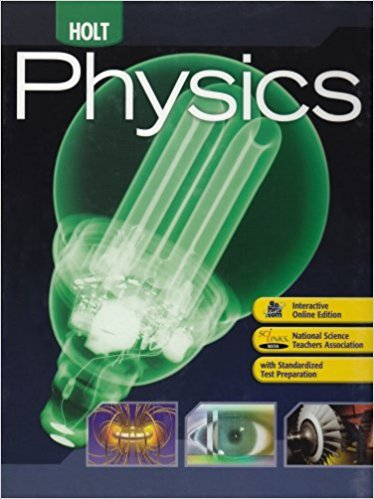×
×

# Indicate which of the following physics symbols denoteISBN: 9780030368165 258

## Solution for problem 1 Chapter 1.3

Holt Physics: Student Edition 2009 | 1st Edition

• Textbook Solutions
• 2901 Step-by-step solutions solved by professors and subject experts
• Get 24/7 help from StudySoup virtual teaching assistantsHolt Physics: Student Edition 2009 | 1st Edition

4 5 0 249 Reviews
16
3
Problem 1

Indicate which of the following physics symbols denote units and which denote variables or quantities. a. C b. c c. C d. t e. T f. T

Step-by-Step Solution:
Step 1 of 3

Physics 2080 Chapter 16 Temperature and Heat Part B January 12, 2016 Amanda Biddlecome 1) Heat -­‐an object with...

Step 2 of 3

Step 3 of 3

##### ISBN: 9780030368165

The full step-by-step solution to problem: 1 from chapter: 1.3 was answered by , our top Physics solution expert on 01/19/18, 04:35PM. The answer to “Indicate which of the following physics symbols denote units and which denote variables or quantities. a. C b. c c. C d. t e. T f. T” is broken down into a number of easy to follow steps, and 27 words. Since the solution to 1 from 1.3 chapter was answered, more than 221 students have viewed the full step-by-step answer. Holt Physics: Student Edition 2009 was written by and is associated to the ISBN: 9780030368165. This textbook survival guide was created for the textbook: Holt Physics: Student Edition 2009, edition: 1. This full solution covers the following key subjects: . This expansive textbook survival guide covers 12 chapters, and 143 solutions.

Unlock Textbook Solution